# Intermediate Organic Chemistry

Figure 3 shows the way in which the orbitals are orientated, you will notice that the p orbitals each point in a different direction x, y and z. The orbitals become 2px , 2py and 2pz . This is to distinguish each electron as unique.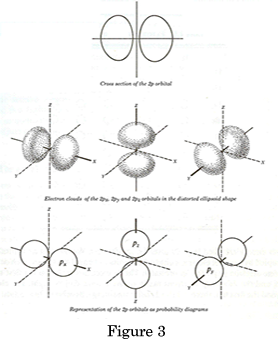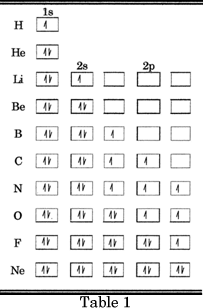Table 1 shows the electronic configuration of the first 10 elements, each electron is unique within the separate orbital; each of the 2 electrons in every orbital has a different spin to make it distinguishable.

If we look at the electronic configuration of carbon: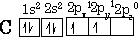We see the configuration of the ground state of the element i.e. the lowest energy state. Carbon does not react in this state it has to undergo a transformation to the excited state by promotion of one of the 2s2 electrons to the 2pz orbital to become: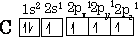The carbon now has 4 orbitals which are half-full, let us now consider how to form a molecule. To do this we need to picture how the atoms come together. For a covalent bond to form, two atoms must be located so that an orbital of one overlaps with that of the other, each orbital to facilitate this merging must contain 1 electron. Each electron must obey the Pauli' rules i.e. have opposite spins. The bond that is formed is now called a molecular orbital.

If we now look at the Hydrogen molecule how does the bonding look in terms of the s orbitals: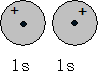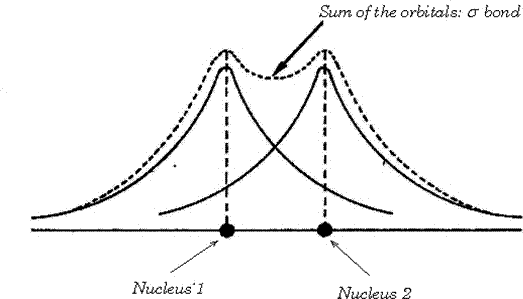These orbitals will now coalesce to form a bond known as a sigma bond (s).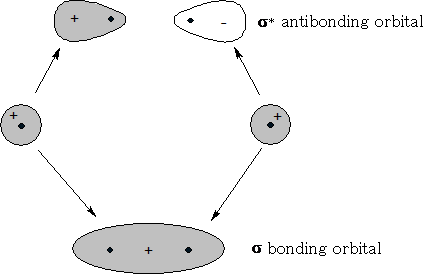Whenever a bonding orbital is formed an equal and opposite antibonding orbital is created see figure 5, the antibonding orbital ( s * ) remains empty in the ground state. The bonding orbital is always lower in energy than the original atoms otherwise a bond would never form.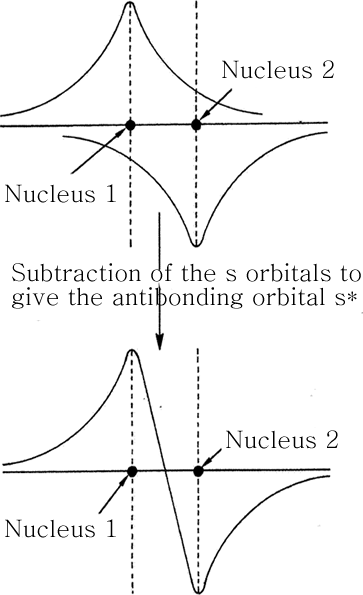Figure 5

Figure 5a shows the way that the electrons are subtracted to form the antibonding orbital. Let us now look at how the p orbitals can also combine to form bonds; consider Fluorine F2 :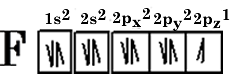The p orbital shown {figure 6} has 1 electron in the 2p z orbital, so this can overlap with the 2p z 1 on the other F atom to form another s bond, this time it will be an end-on overlap of the p orbitals {figure 7}.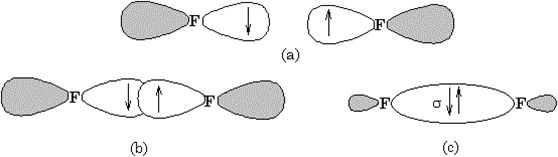Figure 6 and 7

a) separate p orbitals b) overlap c) The s bond orbital

There is also a second way that 2 p orbitals can overlap and that is side on {figure 8}.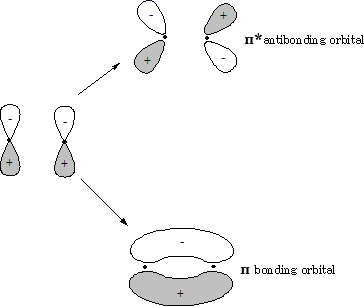Figure 8

What is formed is known as a pi bond (p). You can see that there is an electron cloud above and below the plane of the atoms. This bond is much weaker than the s bond because there is a lot less overlap hence making this p bond reactive. Again an antibonding orbital (p * ) is formed just as we saw in the diagram of the s bond.

If we now look at the carbon atom we can now put together what we have found out about the s and p orbitals!

Page 3 of That Quantum Stuff

References for Quantum Stuff

It was absolutely marvellous working for Pauli. You could ask him anything. There was no worry that he would think a particular question was stupid, since he thought all questions were stupid. Viktor Weisskopf, Amer. J Phys. 45, 422 (1977)

Heisenberg's uncertainty principle: It is impossible to determine simultaneously the position and momentum of an atomic particle. http://www.halexandria.org/dward159.htm

Catenation from the Latin catena which literally means chain so catenation means to form chains.